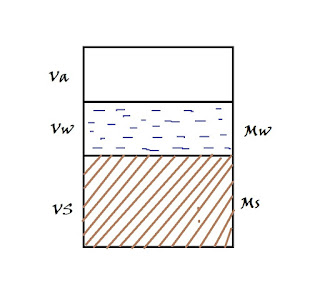# Relationship Between [Void Ratio], Specific Gravity / [Degree of Saturation]

By: Sr vini

In three phase diagrame, we see volumetric relations and volume mass relation, further, thair is Relationship between void ratio specific gravity and degree of saturation.which important in solving the numerical problem and for a better understanding of the chapter.
Fig Show a three phase diagram, the left side of which shows Volume and right side show mass respectively for air-water solid.We know Density ρ = mass / Volume  also this can be written as  mass = Density x Volume
from above we say the mass of water = Density of water x Volume of water = ρw x Vw
Now Mass of solid = density of solid x Volume of Solid, But We don’t know the density of solid
but we know Specific Gravity (G) = Density of solid ρs / Density of Water ρw, from this we can say

Density of solid is ρs = G x ρw
Put these density value in Mass formula M= ρv
our diagram now look like this (second three phase diagram)
Also, we know water content =
Degree of saturation S = volume of water Vw  / volume of voids Vv
also the volume of water Vw = S x Vv

Put all value on star mark equation we get
Now We Know (Vv / Vs) = e        SO our equation will look like
That’s our final Relationship between void ratio specific gravity and degree of saturation